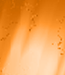## Mapping Key Responses to Likert Task

##### Mapping Key Responses to Likert Task
 Author Message TDeramusGroup: Awaiting Activation Posts: 1, Visits: 13 Hello all.I'm attempting to make a 4 point likert scale in response to pictures presented in an MRI scanner.The participant won't have access to a mouse or keyboard, and our joystick only reads keyboard responses of 6, 7, 8, and 9.I'd like to map the likert to the keyboard so that 1 = 6, 2 = 7, 3 = 8, and 4 = 9, but I don't see exactly how to do that from the element page.Does anyone know how to map button presses to the likert command or would I be better off making response images?-Thomas Daveposted 9 Years Ago ANSWERGroup: Administrators Posts: 12K, Visits: 95K You would not use the element in this particular use case, but set this up using standard etc. elements instead.Something along the lines of/ scalevalue = 0/ columns = [date,time,subject,blocknum,blockcode,trialnum,trialcode,latency,response,values.scalevalue, ...]/ trials = [1=customlikert]/ ontrialend = [if (trial.customlikert.response == 7 || trial.customlikert.response == 77) values.scalevalue = 1]/ ontrialend = [if (trial.customlikert.response == 8 || trial.customlikert.response == 71) values.scalevalue = 2]/ ontrialend = [if (trial.customlikert.response == 9 || trial.customlikert.response == 72) values.scalevalue = 3]/ ontrialend = [if (trial.customlikert.response == 10 || trial.customlikert.response == 73) values.scalevalue = 4]/ stimulusframes = [1=one, two, three, four]/ validresponse = ("6", "7", "8", "9")/ responsemessage = ("6", onehl, 500)/ responsemessage = ("7", twohl, 500)/ responsemessage = ("8", threehl, 500)/ responsemessage = ("9", fourhl, 500)/ items = ("1")/ size = (100px, 100px)/ position = (35%, 60%)/ txcolor = (white)/ txbgcolor = (black)/ items = ("2")/ size = (100px, 100px)/ position = (45%, 60%)/ txcolor = (white)/ txbgcolor = (black)/ items = ("3")/ size = (100px, 100px)/ position = (55%, 60%)/ txcolor = (white)/ txbgcolor = (black)/ items = ("4")/ size = (100px, 100px)/ position = (65%, 60%)/ txcolor = (white)/ txbgcolor = (black)/ items = ("1")/ size = (100px, 100px)/ position = (35%, 60%)/ txcolor = (black)/ txbgcolor = (grey)/ items = ("2")/ size = (100px, 100px)/ position = (45%, 60%)/ txcolor = (black)/ txbgcolor = (grey)/ items = ("3")/ size = (100px, 100px)/ position = (55%, 60%)/ txcolor = (black)/ txbgcolor = (grey)/ items = ("4")/ size = (100px, 100px)/ position = (65%, 60%)/ txcolor = (black)/ txbgcolor = (grey) Edited 9 Years Ago by Dave

#### Merge Selected

Merge into selected topic...

Merge into merge target...

Merge into a specific topic ID...